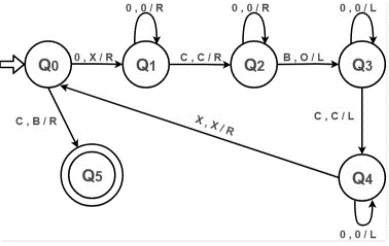# Construct Turing machine for addition

Generally in different finite automata a number is represented in binary format.

Example − 2- 010

3- 011

4- 100

But in case of addition using the Turing machine the system follows a unary format.

Example − 2- 11 or 00

3- 111 or 000

4- 1111 or 0000

In unary format a number is represented

So, let’s consider zero’s to represent any number in the Turing machine for doing addition.

Example

Inputs 4 and 5

4=0000

5=00000

Then 4+5= 0000c00000

Both numbers are separated by a temporary variable c for representing the two numbers

Output: 000000000 =9

The Turing Machine (TM) is as follows −## Explanation

Step 2 − If the symbol is “c” then convert it into blank, move right and jump to step-6.

Step 3 − Ignore 0’s and move towards the right. Ignore “c”, and then move right

Step 4 − Ignore 0’s and move towards the right. Convert a Blank into 0, and then move left

Step 5 − Ignore 0’s and move towards the left.

Step 6 − Ignore “c”, move left.

Step 7 − Ignore 0’s and move towards left.

Step 8 − Ignore an X, move left and jump to step 1.